• Tool for the Field: Polya’s Problem-Solving Method

Back to: Module: Helping Students Do Math

The purpose of this tool for the field is to help paraprofessionals become more familiar with, and practice using, Polya’s four-step problem-solving method.

• Read the section below entitled “Background Information,” and familiarize yourself with the chart of Polya’s four-step problem-solving method.
• Read the example below about Mrs. Byer’s class, and then look over the example of how Polya’s method was used to solve the problem.
• Every person at a party of 12 people said hello to each of the other people at the party exactly once. How many “hellos” were said at the party?
• A new burger restaurant offers two kinds of buns, three kinds of meats, and two types of condiments. How many different burger combinations are possible that have one type of bun, one type of meat, and one condiment type?
• A family has five children. How many different gender combinations are possible, assuming that order matters? (For example, having four boys and then a girl is distinct from having a girl and then four boys.)
• Hillary and Marco are both nurses at the city hospital. Hillary has every fifth day off, and Marco has off every Saturday (and only Saturdays). If both Hillary and Marco had today off, how many days will it be until the next day when they both have off?
• Reflect on your experience. In which types of situations do you think students would find Polya’s method helpful? Are there types of problems for which students would find the method more cumbersome than it is helpful? Can you think of any students who would particularly benefit from a structured problem-solving approach such as Polya’s?## Background Information

Nearly 100 years ago, a man named George Polya designed a four-step method to solve all kinds of problems: Understand the problem, make a plan, execute the plan, and look back and reflect. Because the method is simple and generalizes well, it has become a classic method for solving problems. In fact, the method is applicable to all areas of our lives where we encounter problems—not just math. Although the method appears to be a straightforward method where you start at Step 1, and then go through Steps 2, 3, and 4, the reality is that you will often need to go back and forth through the four steps until you have solved and reflected on a problem.

## Polya’s Problem-Solving Chart: An Example

A version of Polya’s problem-solving chart can be found below, complete with descriptions of each step and an illustration of how the method can be used systematically to solve the following problem:

There are 22 students in Mrs. Byer’s third grade class. Every student is required to either play the recorder or sing in the choir, although students have the option of doing both. Eight of Mrs. Byer’s students chose to play the recorder, and 20 students sing in the choir. How many of Mrs. Byer’s students both play the recorder and sing in the choir?

## Inside this Tool

• Introductory Challenge
• Activity: Does Anyone Know What Math Is?
• Revisiting the Introductory Challenge
• Introductory Challenges
• Activity: The Fennema-Sherman Attitude Scales
• Activity: Asking a Student About His or Her Past Experience with Math
• Activity: Asking Teachers What Teaching Math is Like
• Tool for the Field: Using a Frayer Model for Learning Math Concepts
• Activity: Helping a Child Learn from a Textbook
• Tool for the Field: Using Online Math Resources
• Activity: Helping a Student Learn to use a Calculator
• Tool for the Field: Developing Better Questions Over Time
• Activity: Practice Asking Good Questions
• Activity: Applying Poly’s Method to a Life Decision
• Illustration: Learning Progressions
• Activity: What Do Others Think?
• Tool for the Field: Putting it All Together
• Activity: The Old Guy’s No-Math Test
• Toggle High Contrast
• Toggle Font size## Intermediate Algebra Tutorial 8

• Use Polya's four step process to solve word problems involving numbers, percents, rectangles, supplementary angles, complementary angles, consecutive integers, and breaking even.

Whether you like it or not, whether you are going to be a mother, father, teacher, computer programmer, scientist, researcher, business owner, coach, mathematician, manager, doctor, lawyer, banker (the list can go on and on),  problem solving is everywhere.  Some people think that you either can do it or you can't.  Contrary to that belief, it can be a learned trade.  Even the best athletes and musicians had some coaching along the way and lots of practice.  That's what it also takes to be good at problem solving.

George Polya , known as the father of modern problem solving, did extensive studies and wrote numerous mathematical papers and three books about problem solving.  I'm going to show you his method of problem solving to help step you through these problems.

If you follow these steps, it will help you become more successful in the world of problem solving.

Polya created his famous four-step process for problem solving, which is used all over to aid people in problem solving:

Step 1: Understand the problem.

Step 2:   Devise a plan (translate).

Step 3:   Carry out the plan (solve).

Step 4:   Look back (check and interpret).

Just read and translate it left to right to set up your equation

Since we are looking for a number, we will let

x = a number

*Get all the x terms on one side

*Inv. of sub. 2 is add 2

FINAL ANSWER:  The number is 6.

We are looking for two numbers, and since we can write the one number in terms of another number, we will let

x = another number

ne number is 3 less than another number:

x - 3 = one number

*Inv. of sub 3 is add 3

*Inv. of mult. 2 is div. 2

FINAL ANSWER:  One number is 90. Another number is 87.

When you are wanting to find the percentage of some number, remember that ‘of ’ represents multiplication - so you would multiply the percent (in decimal form) times the number you are taking the percent of.

We are looking for a number that is 45% of 125,  we will let

x = the value we are looking for

FINAL ANSWER:  The number is 56.25.

We are looking for how many students passed the last math test,  we will let

x = number of students

FINAL ANSWER: 21 students passed the last math test.

We are looking for the price of the tv before they added the tax,  we will let

x = price of the tv before tax was added.

*Inv of mult. 1.0825 is div. by 1.0825

FINAL ANSWER: The original price is \$500.

Perimeter of a Rectangle = 2(length) + 2(width)

We are looking for the length and width of the rectangle.  Since length can be written in terms of width, we will let

length is 1 inch more than 3 times the width:

1 + 3 w = length

*Inv. of add. 2 is sub. 2

*Inv. of mult. by 8 is div. by 8

FINAL ANSWER: Width is 3 inches. Length is 10 inches.

Complimentary angles sum up to be 90 degrees.

We are already given in the figure that

x = one angle

5 x = other angle

*Inv. of mult. by 6 is div. by 6

FINAL ANSWER: The two angles are 30 degrees and 150 degrees.

If we let x represent the first integer, how would we represent the second consecutive integer in terms of x ?  Well if we look at 5, 6, and 7 - note that 6 is one more than 5, the first integer.

In general, we could represent the second consecutive integer by x + 1 .  And what about the third consecutive integer.

Well, note how 7 is 2 more than 5.  In general, we could represent the third consecutive integer as x + 2.

Consecutive EVEN integers are even integers that follow one another in order.

If we let x represent the first EVEN integer, how would we represent the second consecutive even integer in terms of x ?   Note that 6 is two more than 4, the first even integer.

In general, we could represent the second consecutive EVEN integer by x + 2 .

And what about the third consecutive even integer?  Well, note how 8 is 4 more than 4.  In general, we could represent the third consecutive EVEN integer as x + 4.

Consecutive ODD integers are odd integers that follow one another in order.

If we let x represent the first ODD integer, how would we represent the second consecutive odd integer in terms of x ?   Note that 7 is two more than 5, the first odd integer.

In general, we could represent the second consecutive ODD integer by x + 2.

And what about the third consecutive odd integer?  Well, note how 9 is 4 more than 5.  In general, we could represent the third consecutive ODD integer as x + 4.

Note that a common misconception is that because we want an odd number that we should not be adding a 2 which is an even number.  Keep in mind that x is representing an ODD number and that the next odd number is 2 away, just like 7 is 2 away form 5, so we need to add 2 to the first odd number to get to the second consecutive odd number.

We are looking for 3 consecutive integers, we will let

x = 1st consecutive integer

x + 1 = 2nd consecutive integer

x + 2  = 3rd consecutive integer

*Inv. of mult. by 3 is div. by 3

FINAL ANSWER: The three consecutive integers are 85, 86, and 87.

We are looking for 3 EVEN consecutive integers, we will let

x = 1st consecutive even integer

x + 2 = 2nd consecutive even integer

x + 4  = 3rd  consecutive even integer

*Inv. of add. 10 is sub. 10

FINAL ANSWER: The ages of the three sisters are 4, 6, and 8.

In the revenue equation, R is the amount of money the manufacturer makes on a product.

If a manufacturer wants to know how many items must be sold to break even, that can be found by setting the cost equal to the revenue.

We are looking for the number of cd’s needed to be sold to break even, we will let

*Inv. of mult. by 10 is div. by 10

To get the most out of these, you should work the problem out on your own and then check your answer by clicking on the link for the answer/discussion for that  problem .  At the link you will find the answer as well as any steps that went into finding that answer.

Practice Problems 1a - 1g: Solve the word problem.

http://www.purplemath.com/modules/translat.htm This webpage gives you the basics of problem solving and helps you with translating English into math.

http://www.purplemath.com/modules/numbprob.htm This webpage helps you with numeric and consecutive integer problems.

http://www.purplemath.com/modules/percntof.htm This webpage helps you with percent problems.

http://www.math.com/school/subject2/lessons/S2U1L3DP.html This website helps you with the basics of writing equations.

http://www.purplemath.com/modules/ageprobs.htm This webpage goes through examples of age problems,  which are like the  numeric problems found on this page.

Go to Get Help Outside the Classroom found in Tutorial 1: How to Succeed in a Math Class for some more suggestions.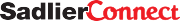• Mathematics
• English Language Arts
• Grammar & Writing
• Virtual Events
• What is Phonics?
• Teaching Grammar
• Vocabulary Games
• What is Virtual Learning?
• Find a Sales Representative
• International Distributors
• International Programs
• Online Catalogs
• Pricing & Ordering Information
• Return Policy
• Terms & Conditions• Counting and Cardinality
• Operations and Algebraic Thinking
• Number and Operations in Base 10
• Measurement and Data
• Math Practices
• Number and Operations Fractions
• Statistics and Probability
• The Number System
• Ratios and Proportional Relationships
• Expressions and Equations
• MP1—Problem Solving
• MP2—Abstract <−>Quantitative
• MP3—Reasoning
• MP4—Modeling
• MP5—Using Tools
• MP6—Precision
• MP7—Structure
• MP8—Repeated Reasoning
• Kindergarten Math Activities
• Professional Development
• Test-Taking Strategies
• Classroom Posters
• Writing In Mathematics

## April 19, 2023 3-5-operations-and-algebraic-thinking , k-2-operations-and-algebraic-thinking , 6-8-expressions-and-equations

Polya’s problem-solving process: finding unknowns elementary & middle school, by: jeff todd.

In this article, we'll explore how a focus on finding “unknowns” in math will lead to active problem-solving strategies for Kindergarten to Grade 8 classrooms. Through the lens of George Polya and his four-step problem-solving heuristic, I will discuss how you can apply the concept of finding unknowns to your classroom. Plus, download my Finding Unknowns in Elementary and Middle School Math Classes Tip Sheet .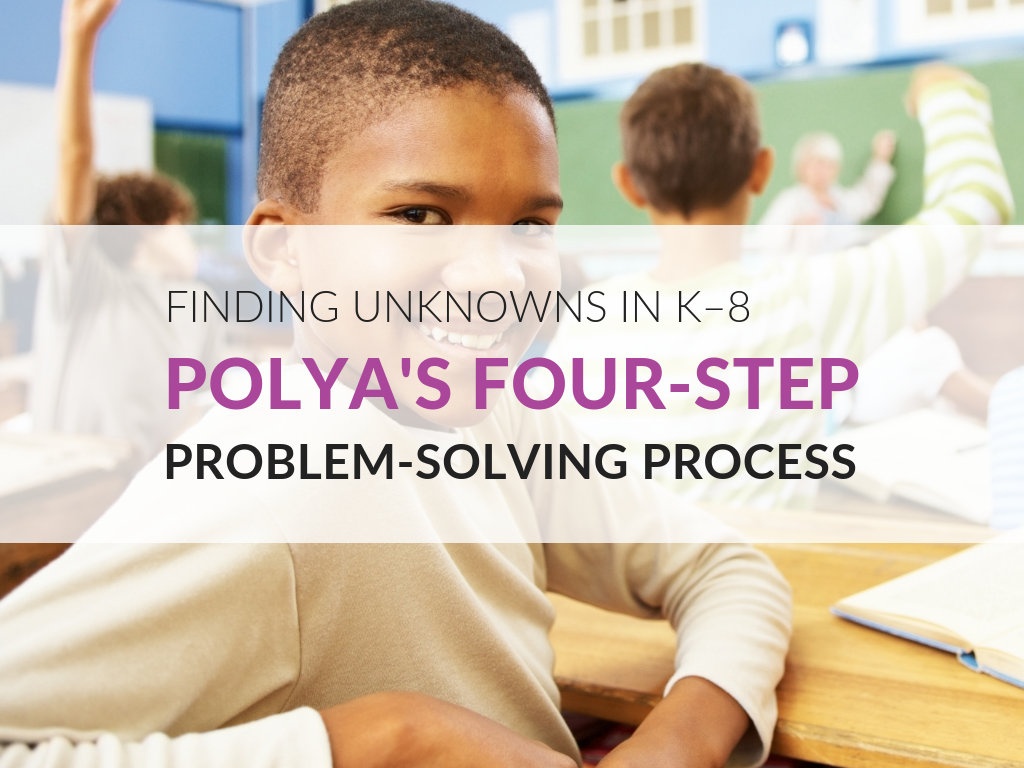It is unfortunate that in the United States mathematics has a reputation for being dry and uninteresting. I hear this more from adults than I do from children—in fact, I find that children are naturally curious about how math works and how it relates to the world around them. It is from adults that they get the idea that math is dry, boring, and unrelated to their lives. Despite what children may or may not hear about math, I focus on making instruction exciting and showing my students that math applicable to their lives.

Problem solving is a fundamental means of developing students' mathematical knowledge and it also shows them that math concepts apply to real-world concepts.

Problem solving is one way I show my students that math relates to their lives! Problem solving is a fundamental means of developing students' mathematical knowledge and it also shows them that math concepts apply to real-world concepts.

## Who Is George Polya?

George Polya was a European-born scholar and mathematician who moved to the U.S in 1940, to work at Stanford University. When considering the his classroom experience of teaching mathematics, he noticed that students were not presented with a view of mathematics that excited and energized them. I know that I have felt this way many times in my teaching career and have often asked: How can I make this more engaging and yet still maintain rigor?

Polya suggested that math should be presented in the light of being able to solve problems. His 1944 book,  How to Solve It  contains his famous four-step problem solving heuristic. Polya suggests that by presenting mathematical thinking as a way to find “unknowns,” it becomes more engaging for students.

He even goes as far as to say that his general four-step problem-solving heuristic can be applied to any field of human endeavor—to any opportunity where a problem exists.

Polya suggested that math should be presented in the light of being able to solve problems...that by presenting mathematical thinking as a way to find “unknowns,” it becomes more engaging for students.

Polya specifically wrote about problem-solving at the high school mathematics level. For those of us teaching students in the elementary and middle school levels, finding ways to apply Polya’s problem-solving process as he intended forces us to rethink the way we teach.

Particularly in the lower grade levels, finding “unknowns” can be relegated to prealgebra and algebra courses in the later grades. Nonetheless, today’s standards call for algebra and algebraic thinking at early grade levels. The  download  for today’s post presents one way you can find unknowns at each grade level.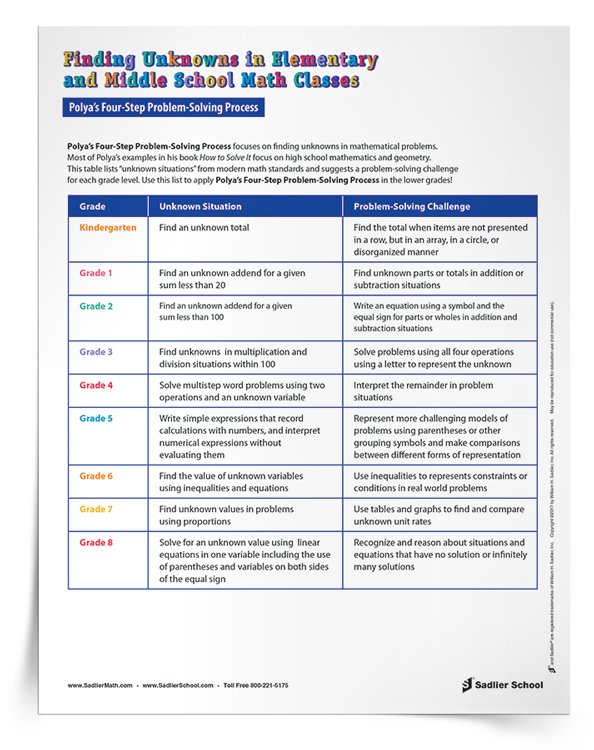## Presenting Mathematics  As A Way To Find "Unknowns" In Real-Life Situations

I would like to share a conversation I had recently with my friend Stu. I have been spending my summers volunteering for a charitable organization in Central America that provides medical services for the poor, runs ESL classes, and operates a Pre-K to Grade 6 school. We were talking about the kind of professional development that I might provide the teachers, and he was intrigued by the thought that we could connect mathematical topics to real life. We specifically talked about the fact that he remembers little or nothing about how to find the area of a figure and never learned in school why it might be important to know about area. Math was presented to him as a set of rules and procedures rather than as a way to find unknowns in real-life situations.

That’s what I am talking about here, and it’s what I believe Polya was talking about. How can we create classrooms where students are able to use their mathematical knowledge to solve problems, whether real-life or purely mathematical?

As Polya noted, there are two ways that mathematics can be presented, either as deductive system of rules and procedures or as an inductive method of making mathematics. Both ways of thinking about mathematics have endured through the centuries, but at least in American education, there has been an emphasis on a procedural approach to math. Polya noticed this in the 1940s, and I think that although we have made progress, there is still an over-emphasis on skill and procedure at the expense of problem-solving and application.

I recently reread Polya’s book. I can’t say that it is an “easy” read, but I would say that it was valuable for me to revisit his own words in order to be sure I understood what he was advocating. As a result, I made the following outline of his problem-solving process and the questions he suggests we use with students.

## Polya's Problem-Solving Process

1. understand the problem, and desiring the solution .

• Restate the problem
• Identify the principal parts of the problem
• Essential questions
• What is unknown?
• What data are available?
• What is the condition?

## 2. Devising a Problem-Solving Plan

• Look at the unknown and try to think of a familiar problem having the same or similar unknown
• Here is a problem related to yours and solved before. Can you use it?
• Can you restate the problem?
• Did you use all the data?
• Did you use the whole condition?

## 3. Carrying Out the Problem-Solving Plan

• Can you see that each step is correct?
• Can you prove that each step is correct?

## 4. Looking Back

• Can you check the result?
• Can you check the argument?
• Can you derive the result differently?
• Can you see the result in a glance?
• Can you use the result, or the method, for some other problem?

## Polya's Suggestions For Helping Students Solve Problems

I also found four suggestions from Polya about what teachers can do to help students solve problems:

Suggestion One In order for students to understand the problem, the teacher must focus on fostering in students the desire to find a solution. Absent this motivation, it will always be a fight to get students to solve problems when they are not sure what to do.

Suggestion Two A second key feature of this first phase of problem-solving is giving students strategies forgetting acquainted with problems.

Suggestion Three Another suggestion is that teachers should help students learn strategies to be able to work toward a better understanding of any problem through experimentation.

Suggestion Four Finally, when students are not sure how to solve a problem, they need strategies to “hunt for the helpful idea.”

Whether you are thinking of problem-solving in a traditional sense (solving computational problems and geometric proofs, as illustrated in Polya’s book) or you are thinking of the kind of problem-solving students can do through STEAM activities, I can’t help but hear echoes of Polya in Standard for Math Practice 1: Make sense of problems and persevere in solving them.

Mathematically proficient students start by explaining to themselves the meaning of a problem and looking for entry points to its solution. They analyze givens, constraints, relationships, and goals. They make conjectures about the form and meaning of the solution and plan a solution pathway rather than simply jumping into a solution attempt. They consider analogous problems, and try special cases and simpler forms of the original problem in order to gain insight into its solution. They monitor and evaluate their progress and change course if necessary.

## In Conclusion

We all know we should be fostering students’ problem-solving ability in our math classes. Polya’s focus on “finding unknowns” in math has wide applicability to problems whether they are purely mathematical or more general.#### IMAGES

1. KS2 maths2. Digital Tools To Teach Problem Solving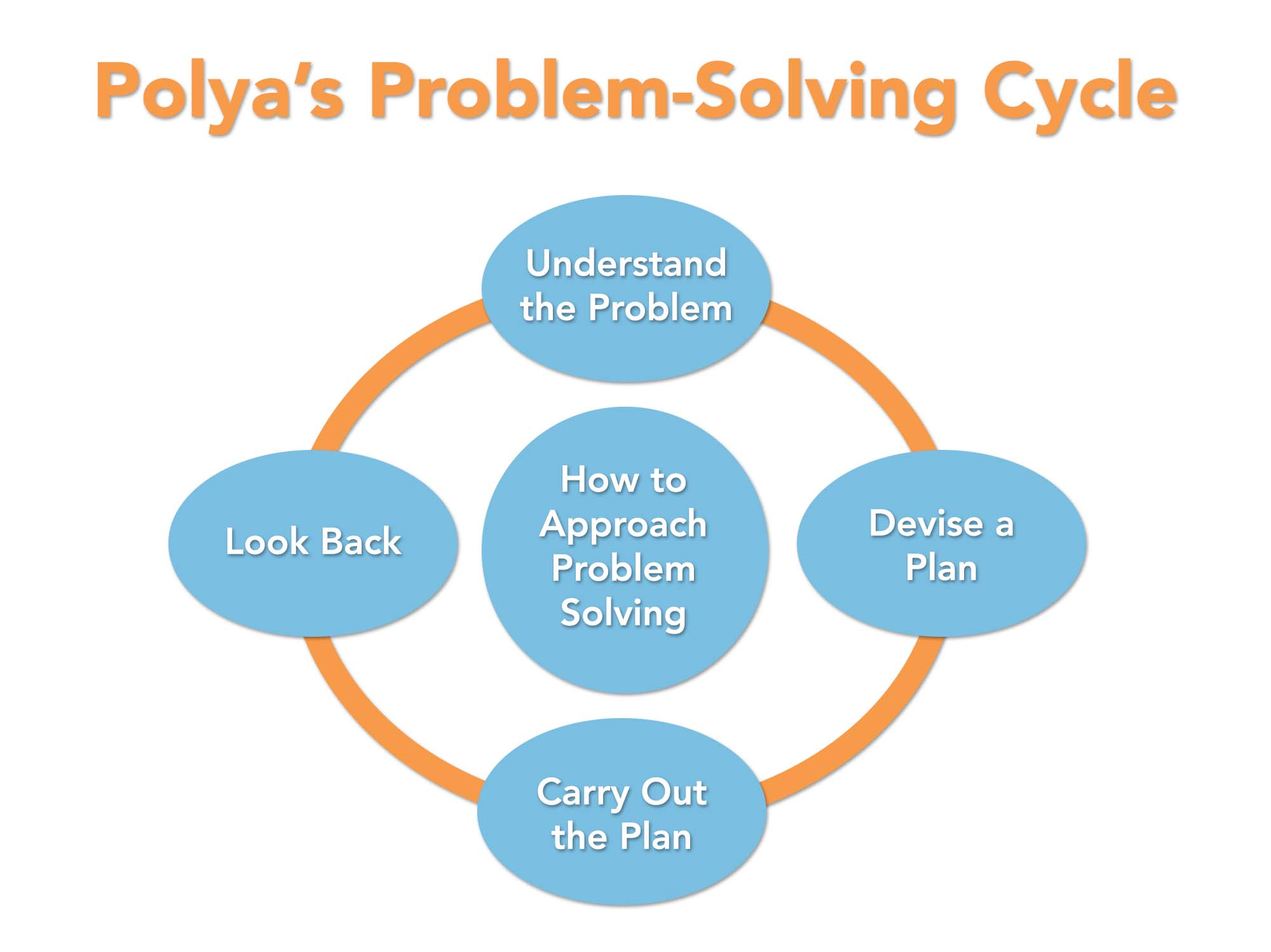3. polya's four step process4. Polya 4 Step Problem Solving5. Polya Problem Solving Strategy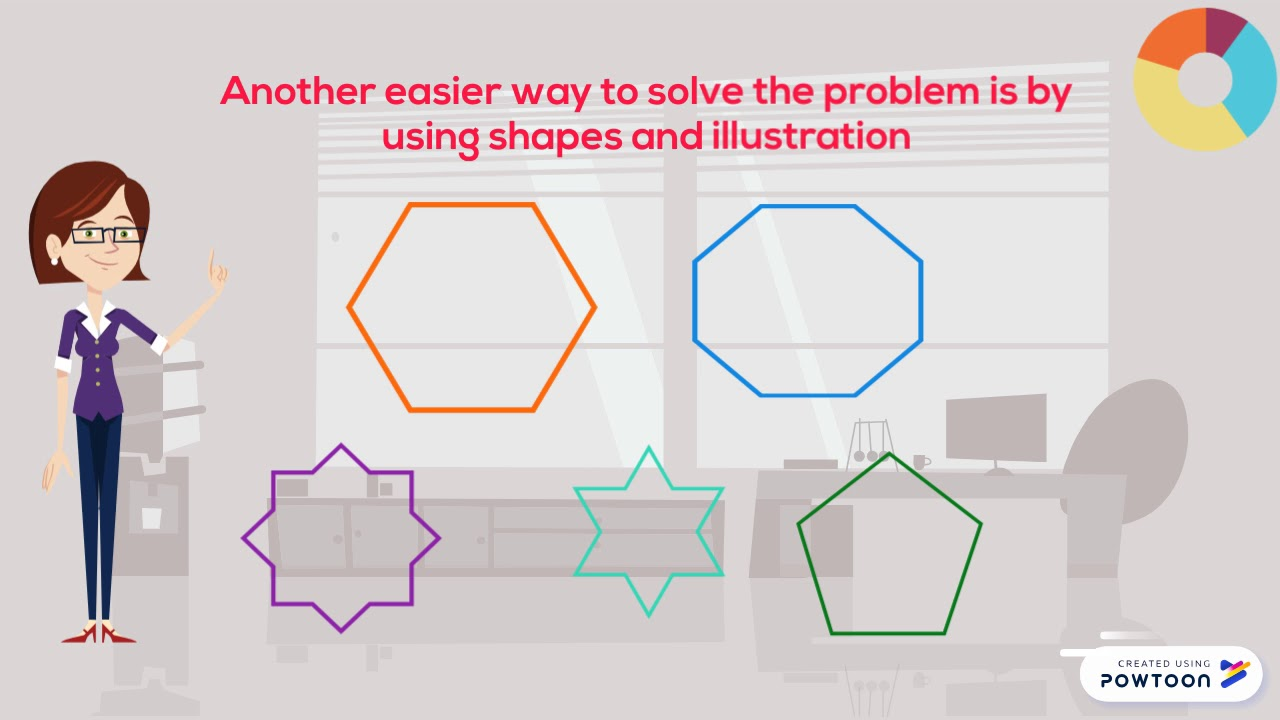6. POLYA's 4 Step Problem Solving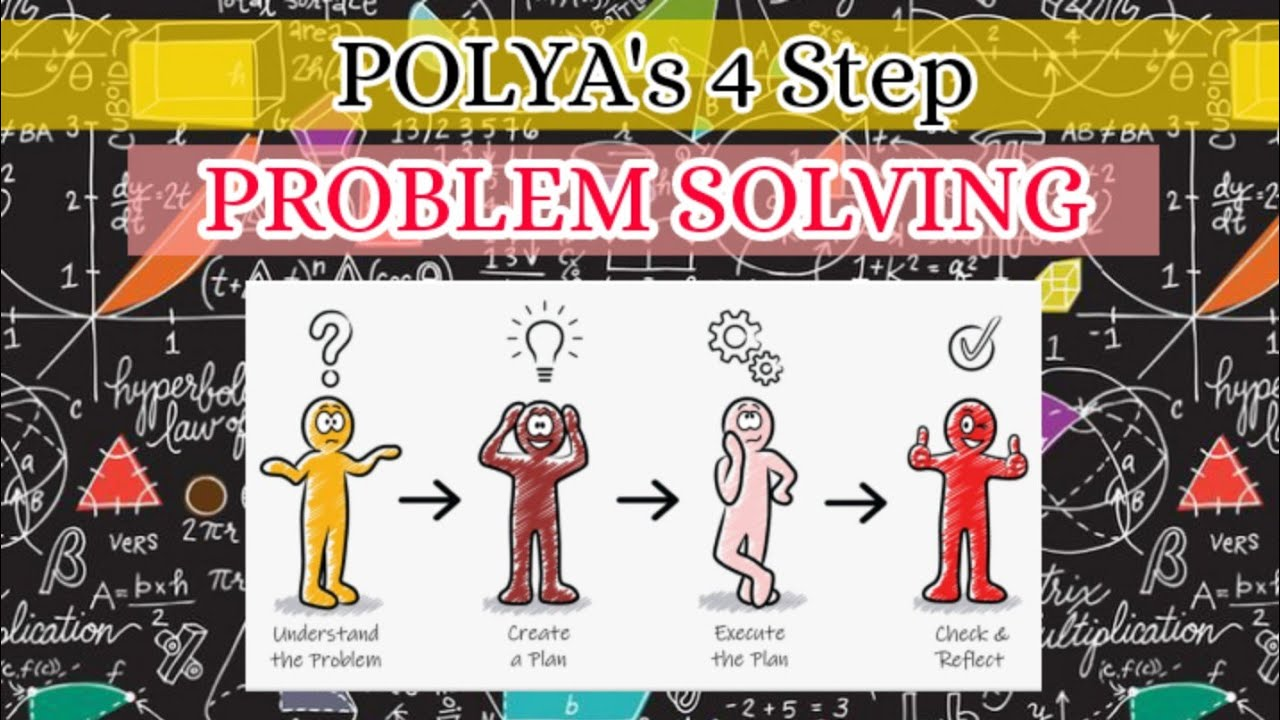#### VIDEO

1. Using Polya's Problem Solving Process to Solve School Management Problems

2. 18th Century Riddle: What Time Is It?

3. Polya's Problem Solving Strategy

4. Solving maths problems:Subtractions

5. Polya's Problem Solving

6. EVT Kids

1. A Step-by-Step Guide to Solving Any Math Problem

Mathematics can be a challenging subject for many students. From basic arithmetic to complex calculus, solving math problems requires logical thinking and problem-solving skills. However, with the right approach and a step-by-step guide, yo...

2. How to Break Down and Solve Complex Math Problems in Your Homework

Math homework can often be a challenging task, especially when faced with complex problems that seem daunting at first glance. However, with the right approach and problem-solving techniques, you can break down these problems into manageabl...

3. Thinking Outside the Box: Creative Approaches to Solve Math Problems

Mathematics can often be seen as a daunting subject, full of complex formulas and equations. Many students find themselves struggling to solve math problems and feeling overwhelmed by the challenges they face.

4. Polya's Problem Solving Techniques

Polya mentions that there are many reasonable ways to solve problems. The

5. Polya's Problem Solving Process

1) Understand the Problem-Make sure you understand what the question is asking and what information will be used

6. Tool for the Field: Polya's Problem-Solving Method

Nearly 100 years ago, a man named George Polya designed a four-step method to solve all kinds of problems: Understand the problem, make a plan, execute the plan

7. Polya's Problem Solving Techniques

Four Steps of Polya's Problem Solving Techniques · Understand the problem · Devise a Plan · Carry out the Plan · Look Back and Reflect.

8. Intermediate Algebra Tutorial 8: Introduction to Problem Solving

... Polya's four steps to problem solving to show students how to solve word problems. Just note that your math teacher or math book may word it

9. Polya's Problem Solving Techniques

Change the problem to use an easier math- ematical structure. Explore what

10. POLYA'S FOUR STEP PROBLEM SOLVING METHOD

Not solving a problem leads to undesirable consequences. “The solution to any problem lies in asking the right questions”. Polya's four step method: A

11. How to Solve It

How to Solve It (1945) is a small volume by mathematician George Pólya describing methods of problem solving. How to Solve It. First edition (publ.

12. Polya's Problem-Solving Process: Finding Unknowns Elementary

Polya suggested that math should be presented in the light of being able to solve problems. His 1944 book, How to Solve It contains his famous four-step problem

13. problem-solving-polya.pdf

the reasons behind what you're saying but you haven't told the readers? • Have you lost track of your audience? Page 36. A math example:.

14. 2.1: George Polya's Four Step Problem Solving Process

2.1: George Polya's Four Step Problem Solving Process ; 1. Guess and test. 11. Solve an equivalent problem. ; 2. Use a variable. 12. Work